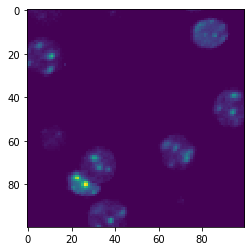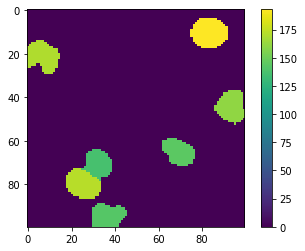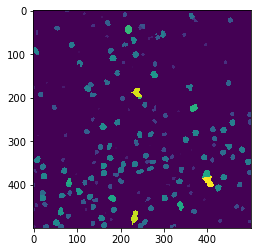# Map area of objects in tiles#

In this notebook, we will segment nuclei in tiles and measure their area. We will then save the resulting are area map again as tiles in a zarr file. This strategy can be used to process data that as a whole does not fit in computer memory.

import zarr
import numpy as np
import pyclesperanto_prototype as cle
from pyclesperanto_prototype import imshow
from numcodecs import Blosc


For demonstration purposes, we use a dataset that is provided by Theresa Suckert, OncoRay, University Hospital Carl Gustav Carus, TU Dresden. The dataset is licensed License: CC-BY 4.0. We are using a cropped version here that was resaved a 8-bit image to be able to provide it with the notebook. You find the full size 16-bit image in CZI file format online.

image = imread('../../data/P1_H_C3H_M004_17-cropped.tif')

# for testing purposes, we crop the image even more.
# comment out the following line to run on the whole 5000x2000 pixels
image = image[1000:1500, 1000:1500]

#compress AND change the numpy array into a zarr array
compressor = Blosc(cname='zstd', clevel=3, shuffle=Blosc.BITSHUFFLE)

# Convert image into zarr array
chunk_size = (100, 100)
zarray = zarr.array(image, chunks=chunk_size, compressor=compressor)

# save zarr to disk
zarr_filename = '../../data/P1_H_C3H_M004_17-cropped.zarr'
zarr.convenience.save(zarr_filename, zarray)


## Object area maps in tiles#

Dask brings built-in support for the zarr file format. We can create dask arrays directly from a zarr file.

zarr_image = da.from_zarr(zarr_filename)
zarr_image

 Array Chunk 244.14 kiB 9.77 kiB (500, 500) (100, 100) 26 Tasks 25 Chunks uint8 numpy.ndarray

We can apply image processing to this tiled dataset directly.

def area_map(image):
"""
Label objects in a binary image and produce a pixel-count-map image.
"""
print("Processing image of size", image.shape)

labels = cle.voronoi_otsu_labeling(image, spot_sigma=3.5)
result = cle.pixel_count_map(labels)

print(result.shape)

return np.asarray(result)


## Testing tiled image processing#

We should test our area mapping algorithm on a single tile. Actually, in a real scenario, the image processing workflow is developed on individual tiles, e.g. in a notebook like this one. As soon as we are sure that the algorithm works, we can apply it to all tiles.

test_image = image[100:200,100:200]

imshow(test_image)

test_result = area_map(test_image)

imshow(test_result, colorbar=True)Processing image of size (100, 100)
(100, 100)## Applying the tiled image processing to a zarr-backed dataset#

Applying the function to our zarr dataset will also result in a dask array.

overlap_width = 30

tile_map = da.map_overlap(area_map, zarr_image, depth=overlap_width, boundary=0)

tile_map

Processing image of size (0, 0)
Processing image of size (1, 1)
(1, 1)
Processing image of size (0, 0)

 Array Chunk 0.95 MiB 39.06 kiB (500, 500) (100, 100) 632 Tasks 25 Chunks float32 numpy.ndarray

Before we can start the computation, we need to deactivate asynchronous execution of operations in pyclesperanto. See also related issue.

cle.set_wait_for_kernel_finish(True)


When we invoke saving the results to disk, the processing will happen on individual tiles.

processed_zarr_filename = '../../data/P1_H_C3H_M004_17-processed.zarr'

tile_map.to_zarr(processed_zarr_filename, overwrite=True)

Processing image of size (160, 160)
Processing image of size (160, 160)
Processing image of size (160, 160)
Processing image of size (160, 160)
Processing image of size (160, 160)
Processing image of size Processing image of size (160, 160)
(160, 160)
Processing image of size (160, 160)
Processing image of size (160, 160)
Processing image of size (160, 160)
(160, 160)
Processing image of size (160, 160)
(160, 160)
(160, 160)
(160, 160)
Processing image of size Processing image of size (160, 160)
(160, 160)
(160, 160)
(160, 160)
(160, 160)
Processing image of size (160, 160)
Processing image of size(160, 160)
(160, 160)
Processing image of size (160, 160)
(160, 160)
Processing image of size (160, 160)
Processing image of size (160, 160)
Processing image of size (160, 160)
(160, 160)
Processing image of size (160, 160)
(160, 160)
Processing image of size (160, 160)
(160, 160)
(160, 160)
(160, 160)
(160, 160)Processing image of sizeProcessing image of size(160, 160)
(160, 160)

(160, 160)
Processing image of size (160, 160)
Processing image of size (160, 160)
(160, 160)
(160, 160)
(160, 160)
(160, 160)
(160, 160)
(160, 160)
(160, 160)
(160, 160)
(160, 160)


zarr_result = da.from_zarr(processed_zarr_filename)

result = zarr_result.compute()